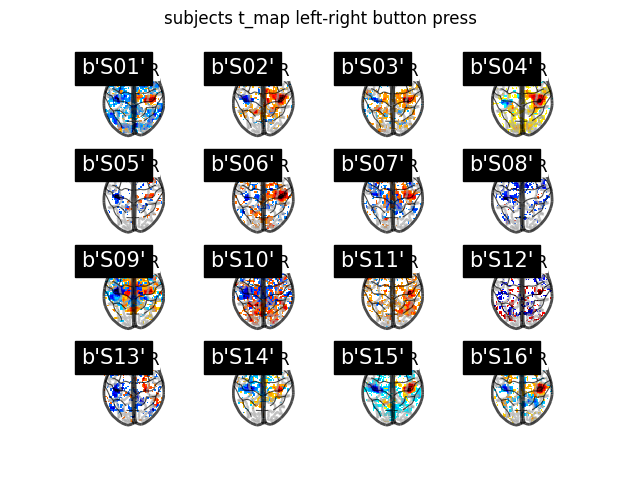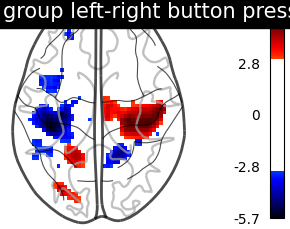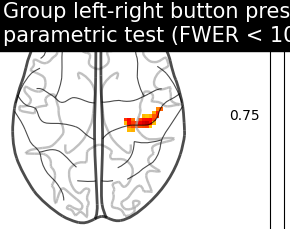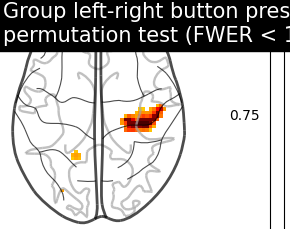# 2.3.5. Second-level fMRI model: one sample test¶

Full step-by-step example of fitting a GLM to perform a second-level analysis (one-sample test) and visualizing the results.

More specifically:

2. A mask of the useful brain volume is computed.

3. A one-sample t-test is applied to the brain maps.

We focus on a given contrast of the localizer dataset: the motor response to left versus right button press. Both at the individual and group level, this is expected to elicit activity in the motor cortex (positive in the right hemisphere, negative in the left hemisphere).

## 2.3.5.1. Fetch dataset¶

We download a list of left vs right button press contrasts from a localizer dataset. Note that we fetch individual t-maps that represent the Bold activity estimate divided by the uncertainty about this estimate.

```from nilearn.datasets import fetch_localizer_contrasts
n_subjects = 16
data = fetch_localizer_contrasts(["left vs right button press"], n_subjects,
get_tmaps=True)
```

Out:

```/home/kshitij/miniconda3/envs/nistats-py36-latest/lib/python3.6/site-packages/numpy/lib/npyio.py:2372: VisibleDeprecationWarning: Reading unicode strings without specifying the encoding argument is deprecated. Set the encoding, use None for the system default.
output = genfromtxt(fname, **kwargs)
```

## 2.3.5.2. Display subject t_maps¶

We plot a grid with all the subjects t-maps thresholded at t = 2 for simple visualization purposes. The button press effect is visible among all subjects.

```from nilearn import plotting
import matplotlib.pyplot as plt
subjects = [subject_data for subject_data in data['ext_vars']]
fig, axes = plt.subplots(nrows=4, ncols=4)
for cidx, tmap in enumerate(data['tmaps']):
plotting.plot_glass_brain(tmap, colorbar=False, threshold=2.0,
title=subjects[cidx],
axes=axes[int(cidx / 4), int(cidx % 4)],
plot_abs=False, display_mode='z')
fig.suptitle('subjects t_map left-right button press')
plt.show()
```Out:

```/home/kshitij/workspace/nistats-org/nistats-repo/nistats-kchawla-pi/examples/03_second_level_models/plot_second_level_one_sample_test.py:49: UserWarning: Matplotlib is currently using agg, which is a non-GUI backend, so cannot show the figure.
plt.show()
```

## 2.3.5.3. Estimate second level model¶

We define the input maps and the design matrix for the second level model and fit it.

```import pandas as pd
second_level_input = data['cmaps']
design_matrix = pd.DataFrame( * len(second_level_input),
columns=['intercept'])
```

Model specification and fit.

```from nistats.second_level_model import SecondLevelModel
second_level_model = SecondLevelModel(smoothing_fwhm=8.0)
second_level_model = second_level_model.fit(second_level_input,
design_matrix=design_matrix)
```

To estimate the contrast is very simple. We can just provide the column name of the design matrix.

```z_map = second_level_model.compute_contrast(output_type='z_score')
```

We threshold the second level contrast at uncorrected p < 0.001 and plot it.

```from scipy.stats import norm
p_val = 0.001
p001_unc = norm.isf(p_val)
display = plotting.plot_glass_brain(
z_map, threshold=p001_unc, colorbar=True, display_mode='z', plot_abs=False,
title='group left-right button press (unc p<0.001)')
plotting.show()
```As expected, we find the motor cortex.

Next, we compute the (corrected) p-values with a parametric test to compare them with the results from a nonparametric test.

```import numpy as np
from nilearn.image import math_img
from nistats.utils import get_data

p_val = second_level_model.compute_contrast(output_type='p_value')
# Correcting the p-values for multiple testing and taking negative logarithm
neg_log_pval = math_img("-np.log10(np.minimum(1, img * {}))"
.format(str(n_voxels)),
img=p_val)
```

Out:

```<string>:1: RuntimeWarning: divide by zero encountered in log10
```

Let us plot the (corrected) negative log p-values for the parametric test.

```cut_coords = 
# Since we are plotting negative log p-values and using a threshold equal to 1,
# it corresponds to corrected p-values lower than 10%, meaning that there
# is less than 10% probability to make a single false discovery
# (90% chance that we make no false discovery at all).
# This threshold is much more conservative than the previous one.
threshold = 1
title = ('Group left-right button press: \n'
'parametric test (FWER < 10%)')
display = plotting.plot_glass_brain(
neg_log_pval, colorbar=True, display_mode='z', plot_abs=False, vmax=3,
cut_coords=cut_coords, threshold=threshold, title=title)
plotting.show()
```Now, we compute the (corrected) p-values with a permutation test.

```from nistats.second_level_model import non_parametric_inference
non_parametric_inference(second_level_input,
design_matrix=design_matrix,
model_intercept=True, n_perm=1000,
two_sided_test=False,
smoothing_fwhm=8.0, n_jobs=1)
```

Let us plot the (corrected) negative log p-values for the nonparametric test.

```title = ('Group left-right button press: \n'
'permutation test (FWER < 10%)')
display = plotting.plot_glass_brain(
display_mode='z', plot_abs=False, cut_coords=cut_coords,
threshold=threshold, title=title)
plotting.show()

# The neg-log p-values obtained with nonparametric testing are capped at 3
# since the number of permutations is 1e3.
# The nonparametric test yields many more discoveries
# and is more powerful than the usual parametric procedure.
```Total running time of the script: ( 0 minutes 16.101 seconds)

Gallery generated by Sphinx-Gallery